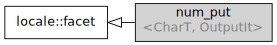/C++

# std::num_put

Defined in header `<locale>`
```template<
class CharT,
class OutputIt = std::ostreambuf_iterator<CharT>
> class num_put;```

Class `std::num_put` encapsulates the rules for formatting numeric values as strings. Specifically, the types `bool`, `long`, `unsigned long`, `long long`, `unsigned long long`, `double`, `long double`, `void*`, and of all types implicitly convertible to these (such as `int` or `float`) are supported. The standard formatting output operators (such as `cout << n;`) use the `std::num_put` facet of the I/O stream's locale to generate text representation of numbers.Inheritance diagram.

### Type requirements

 -`OutputIt` must meet the requirements of LegacyOutputIterator.

### Specializations

Two standalone (locale-independent) full specializations and two partial specializations are provided by the standard library:

Defined in header `<locale>`
`std::num_put<char>` creates narrow string representations of numbers
`std::num_put<wchar_t>` creates wide string representations of numbers
`std::num_put<char, OutputIt>` creates narrow string representations of numbers using custom output iterator
`std::num_put<wchar_t, OutputIt>` creates wide string representations of numbers using custom output iterator

In addition, every locale object constructed in a C++ program implements its own (locale-specific) versions of these specializations.

### Member types

Member type Definition
`char_type` `CharT`
`iter_type` `OutputIt`

### Member functions

 (constructor) constructs a new num_put facet (public member function) (destructor) destructs a num_put facet (protected member function) put invokes `do_put` (public member function)

### Protected member functions

 do_put [virtual] formats a number and writes to output stream (virtual protected member function)

### Member objects

 static std::locale::id id id of the locale (public member object)

### Example

```#include <iostream>
#include <locale>
#include <string>
#include <iterator>

int main()
{
double n = 1234567.89;
std::cout.imbue(std::locale("de_DE"));
std::cout << "Direct conversion to string:\n"
<< std::to_string(n) << '\n'
<< "Output using a german locale:\n"
<< std::fixed << n << '\n'
<< "Output using an american locale:\n";
// use the facet directly
std::cout.imbue(std::locale("en_US.UTF-8"));
auto& f = std::use_facet<std::num_put<char>>(std::cout.getloc());
f.put(std::ostreambuf_iterator<char>(std::cout), std::cout, ' ', n);
std::cout << '\n';
}```

Output:

```Direct conversion to string:
1234567.890000
Output using a german locale:
1.234.567,890000
Output using an american locale:
1,234,567.890000```

 numpunct defines numeric punctuation rules (class template) num_get parses numeric values from an input character sequence (class template) to_string (C++11) converts an integral or floating point value to `string` (function) to_wstring (C++11) converts an integral or floating point value to `wstring` (function)